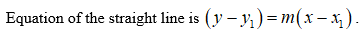# (0,6), m=-1Im supposed to detremine the graph

Question

(0,6), m=-1

Im supposed to detremine the graph

check_circle

Step 1

Consider the given point (0, 6) and the slope m = –1.

First compute the equation of the straight line to determine the graph.

Step 2Step 3

Substitute the point and the slope in t...

### Want to see the full answer?

See Solution

#### Want to see this answer and more?

Solutions are written by subject experts who are available 24/7. Questions are typically answered within 1 hour.*

See Solution
*Response times may vary by subject and question.
Tagged in

### Other